# Python机器学习笔记 使用scikit-learn工具进行PCA降维

+关注继续查看

Python机器学习笔记 使用scikit-learn工具进行PCA降维

在数据处理中，经常会遇到特征维度比样本数量多得多的情况，如果拿到实际工程中去跑，效果不一定好。一是因为冗余的特征会带来一些噪音，影响计算的结果；二是因为无关的特征会加大计算量，耗费时间和资源。所以我们通常会对数据重新变换一下，再跑模型。数据变换的目的不仅仅是降维，还可以消除特征之间的相关性，并发现一些潜在的特征变量。

降维算法由很多，比如PCA ，ICA，SOM，MDS， ISOMAP，LLE等，在此不一一列举。PCA是一种无监督降维算法，它是最常用的降维算法之一，可以很好的解决因变量太多而复杂性，计算量增大的弊端。

PCA算法是一种在尽可能减少信息损失的前提下，找到某种方式降低数据的维度的方法。PCA通常用于高维数据集的探索与可视化，还可以用于数据压缩，数据预处理。

通常来说，我们期望得到的结果，是把原始数据的特征空间（n个d维样本）投影到一个小一点的子空间里去，并尽可能表达的很好（就是损失信息最少）。常见的应用在于模式识别中，我们可以通过减少特征空间的维度，抽取子空间的数据来最好的表达我们的数据，从而减少参数估计的误差。注意，主成分分析通常会得到协方差矩阵和相关矩阵。这些矩阵可以通过原始数据计算出来。协方差矩阵包含平方和向量积的和。相关矩阵与协方差矩阵类似，但是第一个变量，也就是第一列，是标准化后的数据。如果变量之间的方差很大，或者变量的量纲不统一，我们必须先标准化再进行主成分分析。

1，去掉数据的类别特征（label），将去掉后的 d 维数据作为样本

2，计算 d 维的均值向量（即所有数据的每一维向量的均值）

3，计算所有数据的散布矩阵（或者协方差矩阵）

4，计算特征值（e1 , e2 , e3 , .... ed）以及相应的特征向量（lambda1，lambda2，...lambda d）

5，按照特征值的大小对特征向量降序排序，选择前 k 个最大的特征向量，组成 d*k 维的矩阵W（其中每一列代表一个特征向量）

6，运行 d*K 的特征向量矩阵W将样本数据变换成新的子空间。

下面我们看看具体的算法流程：

1） 对有所的样本进行中心化：

2） 计算样本的协方差矩阵

3） 对矩阵进行特征值分解

4） 取出最大的 n' 个特征值对应的特征向量 ，将所有的特征向量标准化后，组成特征向量矩阵W。

5） 对样本集中的每一个样本 ，转化为新的样本

6） 得到输出的样本集

有时候，我们不指定降维后的 n' 的值，而是换种方式，指定一个降维到的主成分比重阈值 t 。这个阈值 t 在 (0 , 1]之间。加入我们的 n个特征值为，则 n' 可以通过下式得到：

PCA算法作为一个非监督学习的降维方法，它只需要特征值分解，就可以对数据进行压缩，去噪。因此在实际场景应用很广泛。为了克服PCA的一些缺点，出现了很多PCA的变种，比如未解决非线性降维的KPCA，还有解决内存限制的增量PCA方法Incremental PCA ，以及解决稀疏数据降维的PCA方法Sparse PCA 等等。

4.1 PCA算法优点
1，仅仅需要以方差衡量信息量，不受数据集以外的因素影响

2，各主成分之间正交，可消除原始数据成分间的相互影响的因素

3，计算方法简单，主要运算时特征值分解，易于实现

4.2 PCA算法缺点
1，主成分各个特征维度的含义具有一定的模糊性，不如原始样本特征的解释性强

2，方差小的非主成分也可能含有对样本差异的重要信息，因降维丢弃可能对后续数据处理有影响。

scikit-learn PCA类介绍
在scikit-learn中，与PCA相关的类都在sklearn.decomposition包中。最常用的PCA类就是sklearn.decomposition.PCA，我们下面主要也会讲解基于这个类的使用的方法。

1
2
3
4
5
6
7
8
9
10
11
12
13
14
15
16
17
18
19
20
21
22
23
24
25
26
27
28
29
30
31
32
33
34
35
36
37
38
39
40
"""
The :mod:sklearn.decomposition module includes matrix decomposition
algorithms, including among others PCA, NMF or ICA. Most of the algorithms of
this module can be regarded as dimensionality reduction techniques.
"""

from .nmf import NMF, non_negative_factorization
from .pca import PCA, RandomizedPCA
from .incremental_pca import IncrementalPCA
from .kernel_pca import KernelPCA
from .sparse_pca import SparsePCA, MiniBatchSparsePCA
from .truncated_svd import TruncatedSVD
from .fastica_ import FastICA, fastica
from .dict_learning import (dict_learning, dict_learning_online, sparse_encode,

                        DictionaryLearning, MiniBatchDictionaryLearning,
SparseCoder)

from .factor_analysis import FactorAnalysis
from ..utils.extmath import randomized_svd
from .online_lda import LatentDirichletAllocation

all = ['DictionaryLearning',

       'FastICA',
'IncrementalPCA',
'KernelPCA',
'MiniBatchDictionaryLearning',
'MiniBatchSparsePCA',
'NMF',
'PCA',
'RandomizedPCA',
'SparseCoder',
'SparsePCA',
'dict_learning',
'dict_learning_online',
'fastica',
'non_negative_factorization',
'randomized_svd',
'sparse_encode',
'FactorAnalysis',
'TruncatedSVD',
'LatentDirichletAllocation']

除了PCA类以外，最常用的PCA相关类还有KernelPCA类，它主要用于非线性数据的降维，需要用到核技巧。因此在使用的时候需要选择合适的核函数并对核函数的参数进行调参。

另外一个常用的PCA相关类是IncrementalPCA类，它主要是为了解决单机内存限制的。有时候我们的样本量可能是上百万+，维度可能也是上千，直接去拟合数据可能会让内存爆掉， 此时我们可以用IncrementalPCA类来解决这个问题。IncrementalPCA先将数据分成多个batch，然后对每个batch依次递增调用partial_fit函数，这样一步步的得到最终的样本最优降维。

此外还有SparsePCA和MiniBatchSparsePCA。他们和上面讲到的PCA类的区别主要是使用了L1的正则化，这样可以将很多非主要成分的影响度降为0，这样在PCA降维的时候我们仅仅需要对那些相对比较主要的成分进行PCA降维，避免了一些噪声之类的因素对我们PCA降维的影响。SparsePCA和MiniBatchSparsePCA之间的区别则是MiniBatchSparsePCA通过使用一部分样本特征和给定的迭代次数来进行PCA降维，以解决在大样本时特征分解过慢的问题，当然，代价就是PCA降维的精确度可能会降低。使用SparsePCA和MiniBatchSparsePCA需要对L1正则化参数进行调参。

下面我们主要基于sklearn.decomposition.PCA来讲解如何使用scikit-learn进行PCA降维。PCA类基本不需要调参，一般来说，我们只需要指定我们需要降维到的维度，或者我们希望降维后的主成分的方差和占原始维度所有特征方差和的比例阈值就可以了。

现在我们对sklearn.decomposition.PCA的主要参数做一个介绍：

1）n_components：这个参数可以帮我们指定希望PCA降维后的特征维度数目。最常用的做法是直接指定降维到的维度数目，此时n_components是一个大于等于1的整数。当然，我们也可以指定主成分的方差和所占的最小比例阈值，让PCA类自己去根据样本特征方差来决定降维到的维度数，此时n_components是一个（0，1]之间的数。当然，我们还可以将参数设置为"mle", 此时PCA类会用MLE算法根据特征的方差分布情况自己去选择一定数量的主成分特征来降维。我们也可以用默认值，即不输入n_components，此时n_components=min(样本数，特征数)。

2）whiten ：判断是否进行白化。所谓白化，就是对降维后的数据的每个特征进行归一化，让方差都为1.对于PCA降维本身来说，一般不需要白化。如果你PCA降维后有后续的数据处理动作，可以考虑白化。默认值是False，即不进行白化。

3）svd_solver：即指定奇异值分解SVD的方法，由于特征分解是奇异值分解SVD的一个特例，一般的PCA库都是基于SVD实现的。有4个可以选择的值：{‘auto’, ‘full’, ‘arpack’, ‘randomized’}。randomized一般适用于数据量大，数据维度多同时主成分数目比例又较低的PCA降维，它使用了一些加快SVD的随机算法。 full则是传统意义上的SVD，使用了scipy库对应的实现。arpack和randomized的适用场景类似，区别是randomized使用的是scikit-learn自己的SVD实现，而arpack直接使用了scipy库的sparse SVD实现。默认是auto，即PCA类会自己去在前面讲到的三种算法里面去权衡，选择一个合适的SVD算法来降维。一般来说，使用默认值就够了。

除了这些输入参数外，有两个PCA类的成员值得关注。第一个是explained_variance_，它代表降维后的各主成分的方差值。方差值越大，则说明越是重要的主成分。第二个是explained_variance_ratio_，它代表降维后的各主成分的方差值占总方差值的比例，这个比例越大，则越是重要的主成分。,

components_ ：返回具有最大方差的成分。

explained_variance_ratio_ ：返回所保留的 n 个成分各自的方差百分比

n_components_：返回所保留的成分个数 n

mean_ ：

noise_variance_ ：

1） fit(X, y=None) fit() 可以说是scikit-learn中通用的方法，每个需要训练的算法都会有fit()方法，他其实就是算法中的“训练”这一步骤。因为PCA是无监督学习算法，此处y自然等于None。fit(X)，表示用数据 X 来训练PCA模型。函数返回值：调用fit方法的对象本身。比如pca.fit(X)，表示用X对pca这个对象进行训练。

2）fit_transform(X) 用X来训练PCA模型，同时返回降维后的数据， NewX = pca.fit_transform(X)。NewX就是降维后的数据。

3）inverse_transform() 将降维后的数据转换成原始数据，X = pca.inverse_transform(NewX)

4）tranform(X) 将数据X转换成降维后的数据。当模型训练好后，对于新输入的数据，都可以用transform 方法来降维。

此外，还有get_covariance()，get_precision()，get_params(deep = True)，score(X, y =True) 等方法。

下面我们用一个实例来学习一下scikit-learn中的PCA类使用。

1，首先生成随机数据并可视化
1
2
3
4
5
6
7
8
9
10
11
12
13
14
15
16
import numpy as np
import matplotlib.pyplot as plt
from mpl_toolkits.mplot3d import Axes3D

# make_blobs会根据用户指定的特征数量，中心点数量，范围等来生成几类数据

from sklearn.datasets.samples_generator import make_blobs

# centers表示中心点 cluster_std表示分布的标准差

X, y = make_blobs(n_samples=10000, n_features=3, centers=[[3, 3, 3], [0, 0, 0], [1, 1, 1],

            [2, 2, 2]], cluster_std=[0.2, 0.1, 0.2, 0.2], random_state=9)

fig = plt.figure()
ax = Axes3D(fig, rect=[0, 0, 1, 1], elev=30, azim=20)
plt.scatter(X[:, 0], X[:, 1], X[:, 2], marker='o')
plt.show()
三维数据的分布图如下：

1
2
3
4
5
6
from sklearn.decomposition import PCA

pca = PCA(n_components=3)
pca.fit(X)
print(pca.explained_variance_ratio_)
print(pca.explained_variance_)
输出结果如下：

1
2
3
[0.98318212 0.00850037 0.00831751]

[3.78521638 0.03272613 0.03202212]
可以看出投影后三个特征维度的方差比例大约为98.3% 0.8% 0.8% 。投影后第一个特征占了绝大多数的主成分比例。

现在我们来进行降维，从三维降到二维，代码如下：

1
2
3
4
5
6
from sklearn.decomposition import PCA

pca = PCA(n_components=2)
pca.fit(X)
print(pca.explained_variance_ratio_)
print(pca.explained_variance_)

输出结果如下：

1
2
3
[0.98318212 0.00850037]

[3.78521638 0.03272613]
这个结果其实可以预料，因为上面三个投影后的特征维度的方差分别为：[3.78521638 0.03272613 0.03202212] ，投影到二维后选择的肯定是钱两个特征，而抛弃第三个特征。

为了由直观的认识，我们看看此时转化后的数据分布，代码如下：

1
2
3
X_new = pca.transform(X)
plt.scatter(X_new[:, 0], X_new[:, 1],marker='o')
plt.show()
（报错待学习）

现在我们 看看不直接指定降维的维度，而指定降维后的主成分方差和比例。

1
2
3
4
5
pca = PCA(n_components=0.95)
pca.fit(X)
print(pca.explained_variance_ratio_)
print(pca.explained_variance_)
print(pca.n_components_)
上面我们指定了主成分至少占95% ，输出如下：

1
2
3
[0.98318212]
[3.78521638]
1
可见只有第一个投影特征被保留，这也很好理解，我们的第一个主成分占投影特征的方差比例高达98%。只选择这一个特征维度便可以满足95%的阈值。我们现在选择阈值99%看看，代码如下：

1
2
3
4
5
pca = PCA(n_components = 0.99)
pca.fit(X)
print(pca.explained_variance_ratio_)
print(pca.explained_variance_)
print(pca.n_components_)
此时输出的结果：

1
2
3
[0.98318212 0.00850037]
[3.78521638 0.03272613]
2
这个结果也很好理解，因为我们第一个主成分占了98.3%的方差比例，第二个主成分占了0.8%的方差比例，两者一起可以满足我们的阈值。

最后我们看看让MLE算法自己选择降维维度的效果，代码如下：

1
2
3
4
5
pca = PCA(n_components = 'mle')
pca.fit(X)
print(pca.explained_variance_ratio_)
print(pca.explained_variance_)
print(pca.n_components_)
输出结果如下：

1
2
3
[0.98318212]
[3.78521638]
1
可见由于我们的数据的第一个投影特征的方差占比高达98.3%，MLE算法只保留了我们的第一个特征。

IRIS数据集是常见的分类试验数据集，也成为鸢尾花数据集，是一类多重变量分析的数据集。数据集包含150个数据集，分为三类，没类50个数据，每个数据包含4个特征。可以通过花萼长度，花萼宽度，花瓣长度，花瓣宽度（sepal length，sepal width ，petal length ，petal width ）4个特征预测鸢尾花卉属于（Setosa，Versicolour，Virginica）三个种类中的哪一类。

1，选取三个特征查看数据分布情况
选取三个特征的原因是人对三维空间比较有概念

1
2
3
4
5
6
7
8
9
10
11
12
13
14
15
16
17
18
19

# coding:utf-8

import matplotlib.pyplot as plt
from mpl_toolkits.mplot3d import Axes3D
from sklearn import datasets

X =data['data']
y =data['target']
ax = Axes3D(plt.figure())
for c, i, target_name in zip('rgb', [0, 1, 2], data.target_names):

ax.scatter(X[y==i, 0], X[y==i, 2], c=c, label=target_name)


ax.set_xlabel(data.feature_names)
ax.set_xlabel(data.feature_names)
ax.set_xlabel(data.feature_names)
ax.set_title('Iris')
plt.legend()
plt.show()
在X[y==i ,0], X[y==i, 1], X[y==i,2]中，通过0，1，2选择了三个特征

可以看出：红色的Setosa离得远。

2，选取两个特征查看数据分布情况
1
2
3
4
5
6
7
8
9
10
11
12
13
14
15
16
17
18

# coding:utf-8

import matplotlib.pyplot as plt
from mpl_toolkits.mplot3d import Axes3D
from sklearn import datasets

X =data['data']
y =data['target']
ax = Axes3D(plt.figure())
for c, i, target_name in zip('rgb', [0, 1, 2], data.target_names):

ax.scatter(X[y==i, 0], X[y==i, 1], c=c, label=target_name)


ax.set_xlabel(data.feature_names)
ax.set_xlabel(data.feature_names)
ax.set_title('Iris')
plt.legend()
plt.show()
结果如下：

可见：利用特征子集，红色的setosa仍然是线性可分的。

3，利用PCA降维，降到二维
1
2
3
4
5
6
7
8
9
10
11
12
13
14
15
16
17
18
19
20

# coding:utf-8

import matplotlib.pyplot as plt
from mpl_toolkits.mplot3d import Axes3D
from sklearn import datasets
from sklearn.decomposition import PCA

X =data['data']
y =data['target']
pca = PCA(n_components=2)
X_p = pca.fit(X).transform(X)
ax = plt.figure()
for c, i, target_name in zip('rgb', [0, 1, 2], data.target_names):

plt.scatter(X_p[y==i, 0], X_p[y==i, 1], c=c, label=target_name)


plt.xlabel('Dimension1')
plt.ylabel('Dimension2')
plt.title('Iris')
plt.legend()
plt.show()
结果如图：

4，利用pandas库查看数据分析
pandas数据可以理解为一种结构化的表格数据

1
2
3
4
5
6
7
8
9
10
11
12
13
14
15
16
17
18
19

# coding:utf-8

import matplotlib.pyplot as plt
from mpl_toolkits.mplot3d import Axes3D
from sklearn import datasets
from sklearn.decomposition import PCA
import pandas as pd
import seaborn

X =data['data']
y =data['target']
a = pd.DataFrame(X, columns=data.feature_names)

# 随机打印一下看看数据

print(a.sample(5))

# 箱图，看数据范围

seaborn.boxplot(data= a)
plt.plot(a)
plt.legend(data.feature_names)
plt.show()
箱型图：

折线图：

5，Incremental PCA
当要分解的数据集太大而无法放入内存时，增量主成分分析（IPCA）通常用作主成分分析（PCA）的替代。IPCA主要是为了解决单机内存限制的，有时候我们的样本量可能是上百万+，维度可能也是上千，直接去拟合数据可能会让内存爆掉，此时我们可以使用Incremental PCA类来解决这个问题。

IPCA 是将数据分成多个batch，然后对每个batch依次递增调用partial_fit函数，这样一步步的得到最终的样本最优降维。

此处示例用作视觉检查，IPCA能够找到类似的数据投影到PCA，而一次只处理几个样本。还可以被视为“玩具示例”。

1
2
3
4
5
6
7
8
9
10
11
12
13
14
15
16
17
18
19
20
21
22
23
24
25
26
27
28
29
30
31
32
33
34
import numpy as np
import matplotlib.pyplot as plt

from sklearn.decomposition import PCA , IncrementalPCA

X = iris.data
y = iris.target

n_components = 2
ipca = IncrementalPCA(n_components=n_components, batch_size=10)
X_ipca = ipca.fit_transform(X)

pca = PCA(n_components=n_components)
X_pca = pca.fit_transform(X)

colors = ['navy', 'turquoise', 'darkorange']

for X_trainsformed, title in [(X_ipca, "Incremental PCA"), (X_pca, "PCA")]:

plt.figure(figsize=(8, 8))
for color, i ,target_name in zip(colors, [0,1,2], iris.target_names):
plt.scatter(X_trainsformed[y == i, 0], X_trainsformed[y ==i, 1],
color=color, lw=2, label=target_name)

if "Incremental" in title:
err = np.abs(np.abs(X_pca) - np.abs(X_ipca)).mean()
plt.title(title + 'of iris dataset\nMean absolute unsigned error%.6f'%err)
else:
plt.title(title + 'of iris dataset')
plt.axis([-4, 4, -1.5, 1.5])


plt.show()

6，Kernel PCA
下面示例可以显示Kernel PCA能够找到使数据线性可分的数据投影

1
2
3
4
5
6
7
8
9
10
11
12
13
14
15
16
17
18
19
20
21
22
23
24
25
26
27
28
29
30
31
32
33
34
35
36
37
38
39
40
41
42
43
44
45
46
47
48
49
50
51
52
53
54
55
56
57
58
59
60
61
62
63
64
65
66
import numpy as np
import matplotlib.pyplot as plt

from sklearn.decomposition import PCA, KernelPCA
from sklearn.datasets import make_circles
np.random.seed(0)

X, y = make_circles(n_samples=400, factor=0.3, noise=0.05)

kpca = KernelPCA(kernel='rbf', fit_inverse_transform=True, gamma=10)
X_kpca = kpca.fit_transform(X)
X_back = kpca.inverse_transform(X_kpca)
pca = PCA()
X_pca = pca.fit_transform(X)

# Plot results

plt.figure()
plt.subplot(2, 2, 1, aspect='equal')
plt.title("Original space")
reds = y == 0
blues = y == 1

plt.scatter(X[reds, 0], X[reds, 1], c="red",

        s=20, edgecolor='k')

plt.scatter(X[blues, 0], X[blues, 1], c="blue",

        s=20, edgecolor='k')

plt.xlabel("$x_1$")
plt.ylabel("$x_2$")

X1, X2 = np.meshgrid(np.linspace(-1.5, 1.5, 50), np.linspace(-1.5, 1.5, 50))
X_grid = np.array([np.ravel(X1), np.ravel(X2)]).T

# projection on the first principal component (in the phi space)

Z_grid = kpca.transform(X_grid)[:, 0].reshape(X1.shape)
plt.contour(X1, X2, Z_grid, colors='grey', linewidths=1, origin='lower')

plt.subplot(2, 2, 2, aspect='equal')
plt.scatter(X_pca[reds, 0], X_pca[reds, 1], c="red",

        s=20, edgecolor='k')

plt.scatter(X_pca[blues, 0], X_pca[blues, 1], c="blue",

        s=20, edgecolor='k')

plt.title("Projection by PCA")
plt.xlabel("1st principal component")
plt.ylabel("2nd component")

plt.subplot(2, 2, 3, aspect='equal')
plt.scatter(X_kpca[reds, 0], X_kpca[reds, 1], c="red",

        s=20, edgecolor='k')

plt.scatter(X_kpca[blues, 0], X_kpca[blues, 1], c="blue",

        s=20, edgecolor='k')

plt.title("Projection by KPCA")
plt.xlabel(r"1st principal component in space induced by $\phi$")
plt.ylabel("2nd component")

plt.subplot(2, 2, 4, aspect='equal')
plt.scatter(X_back[reds, 0], X_back[reds, 1], c="red",

        s=20, edgecolor='k')

plt.scatter(X_back[blues, 0], X_back[blues, 1], c="blue",

        s=20, edgecolor='k')

plt.title("Original space after inverse transform")
plt.xlabel("$x_1$")
plt.ylabel("$x_2$")

plt.subplots_adjust(0.02, 0.10, 0.98, 0.94, 0.04, 0.35)

plt.show()
结果展示：

（对于代码功能的注释，通过__doc__ 可以输出）

每个对象都会有一个__doc__属性，用于描述该对象的作用。在一个模块被import时，其文件中的某些特征的字符串会被python解释器保存在相应对象的__doc__属性中。

比如，一个模块有模块的__doc__，一个class或者function也有其对应的__doc__属性。在python中，一个模块其实就是一个 .py 文件。在文件中特殊的地方书写的字符串就是所谓的docstrings。就是将被放在__doc__的内容。这个“特殊的地方 “ 包括：

1， 一个文件任何一条可执行的代码之前 # 模块的__doc__

2， 一个类，在类定于语句后，任何可执行代码前 # 类的 doc

3，一个函数，在函数定于语句后，任何可执行代码前 # 函数的__doc__

1
2
3
4
5
6
7
8
9
10
11
12
13
14
15

# use doc 属性

class MyClass:

'string.'

def printSay(self):
'print say welcome to you.'
print('say welcome to you.')


print(MyClass.__doc__)
print(MyClass.printSay.__doc__)

# 输出结果

string.
print say welcome to you.

https://www.cnblogs.com/pinard/p/6239403.html3 0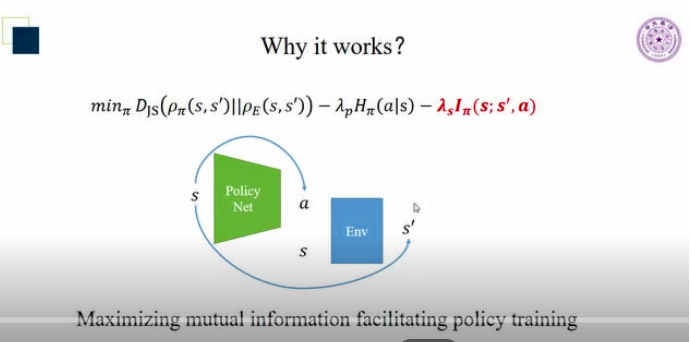NeurIPS 2019分享：清华大学孙富春组提出全新模仿学习理论

1 05 02 01997年，国际象棋名家卡斯帕罗夫象棋对弈IBM超级电脑深蓝，最后结果是大师输了。 2016年，世界顶级围棋高手李世石与AI围棋对决，最后竟以1:4惨败于谷歌阿尔法狗。 今年4月，AI电竞团队OpenAI Five与人类战队对决《dota》，2:0 完胜世界冠军OG战队。 人工智能凭什么能够战胜人类?答案是AI背后的超级算力。AI通过算力处理大量的相关数据，并以神经网络不断学习成长，最终获得技能，战胜人类选手。 算力经济，算力时代，算力改变世界，算力驱动未来，算力…… 现在很多领域都在谈论算力，到底什么是算力？
3 02018年下半年开始的这场互联网裁员潮，延续至今，且还在继续。保守估计，目前裁员涉及的知名企业或已超过40家，其中不乏新晋互联网小巨头TMD中的美团和滴滴。就连BAT中的腾讯、百度都没能避免，阿里虽已对裁员消息辟谣，是否真的裁员只有员工自己清楚了。
3 0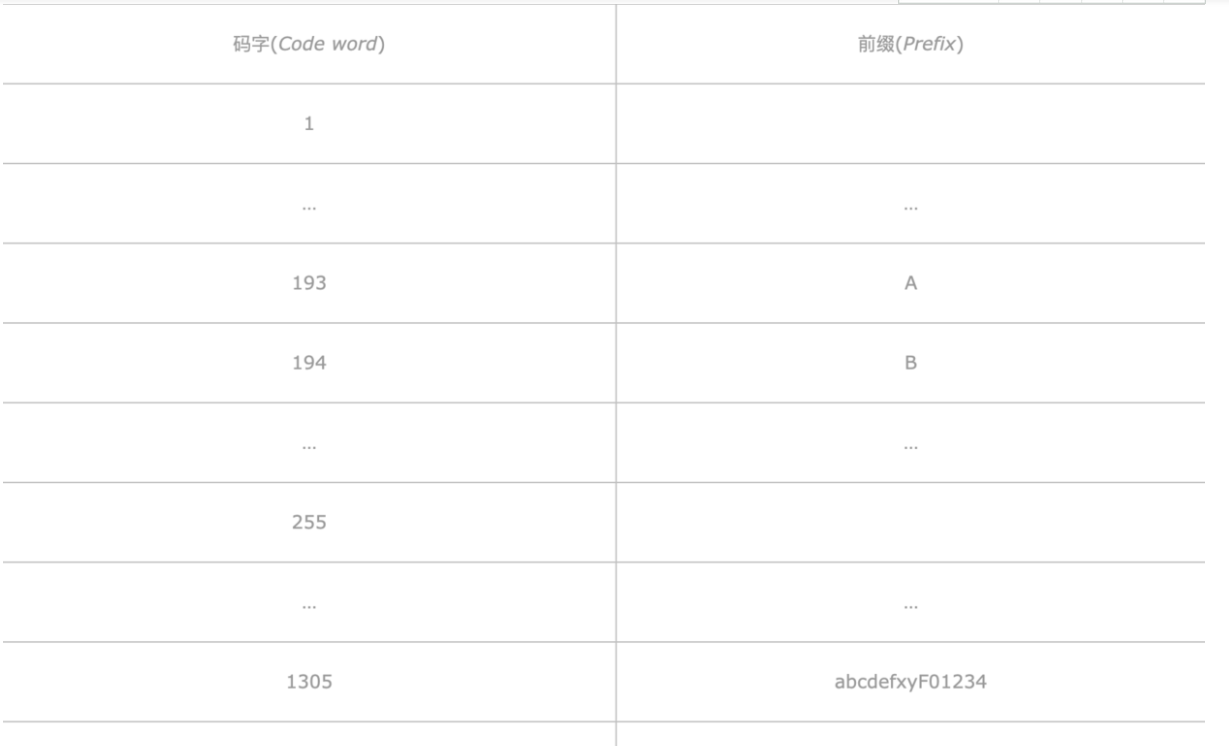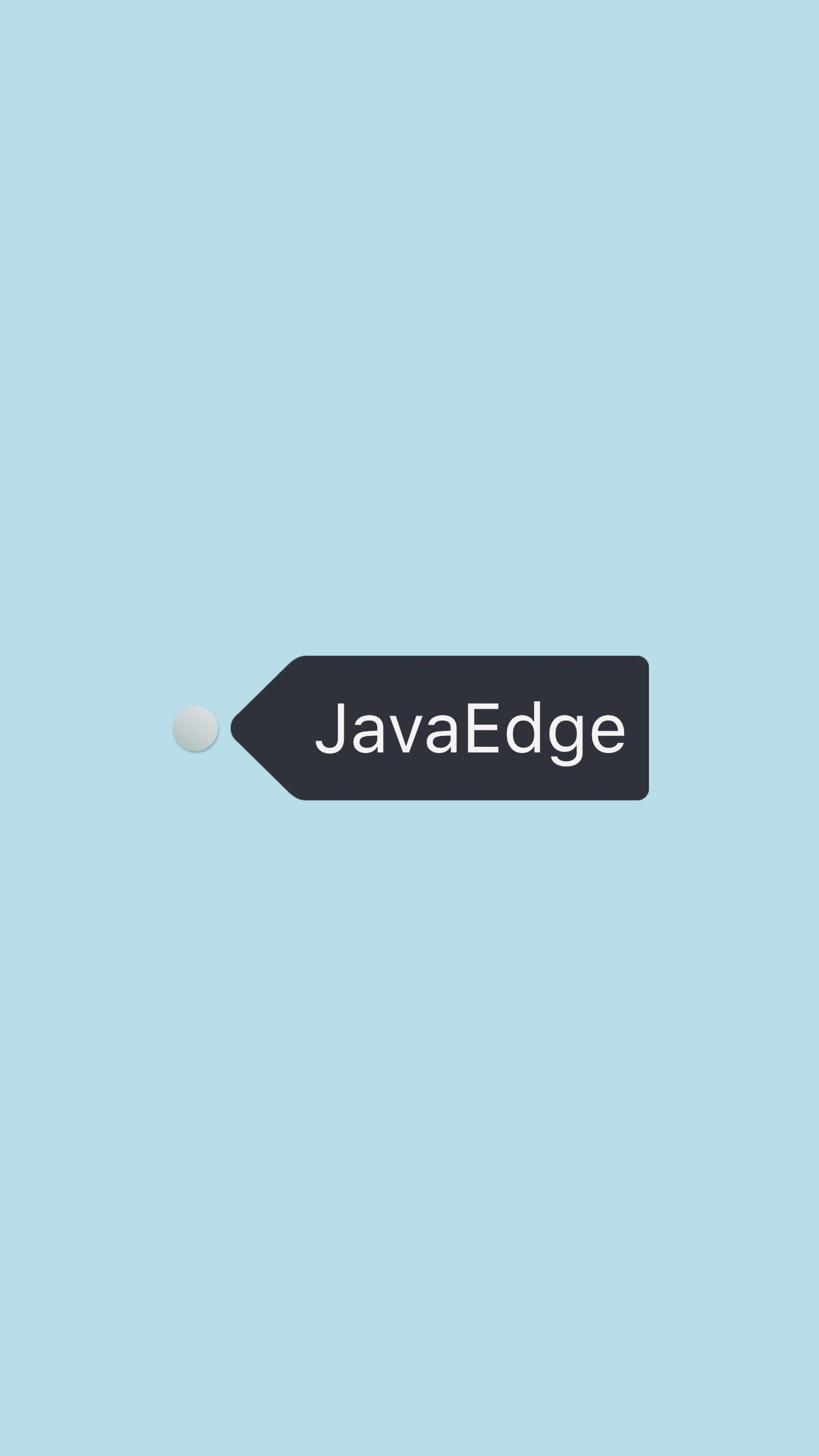3 0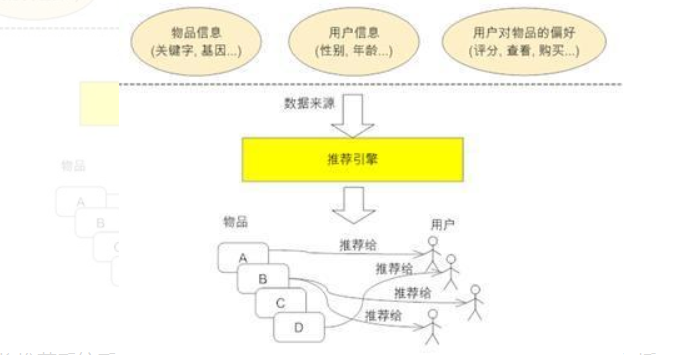3 0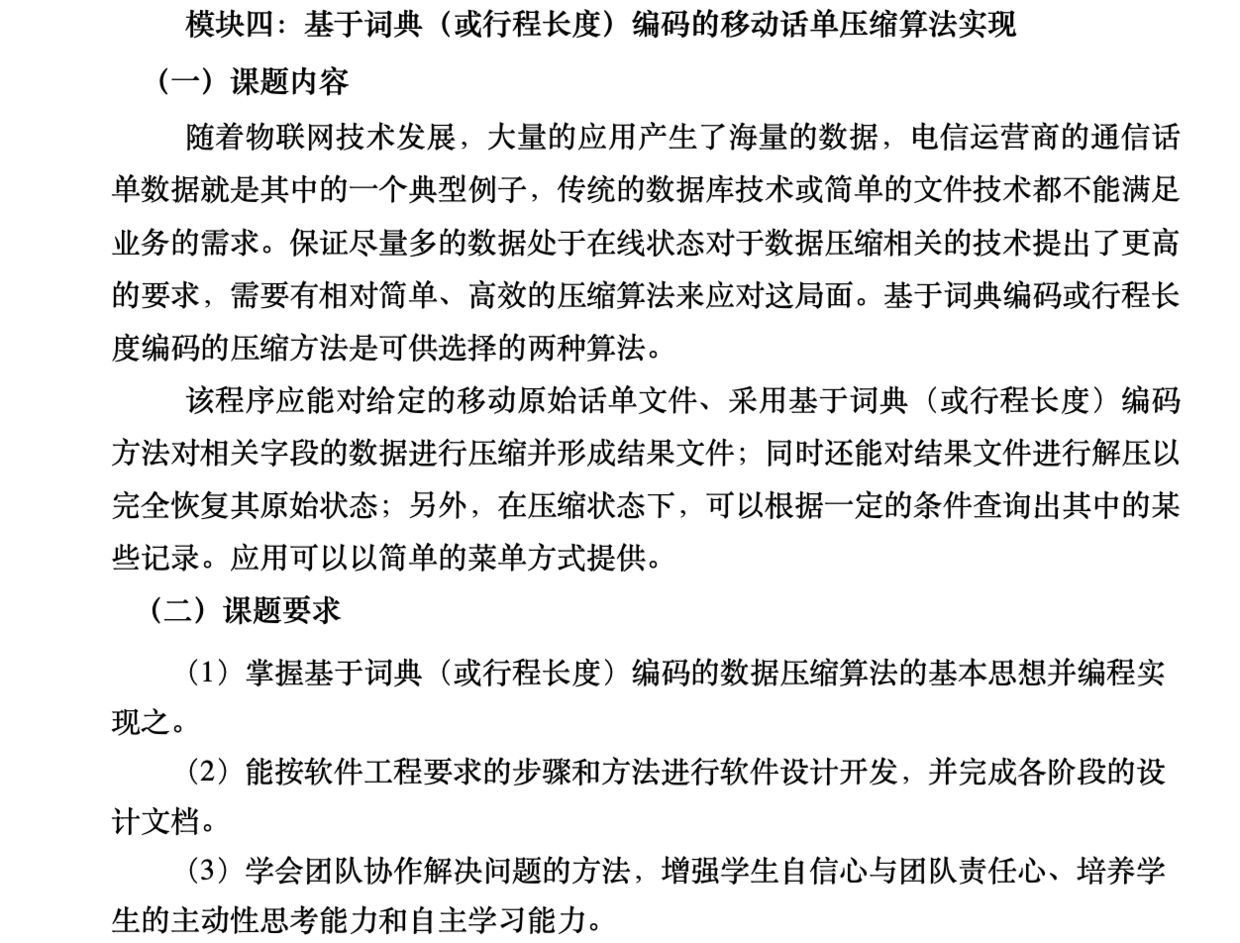3 0

158

0

《SaaS模式云原生数据仓库应用场景实践》

《看见新力量：二》电子书